Function（Under Construction!）

Function

```finite step function ---->  cycle finding
infinite step function ---->  dynamic system / fractal
```

Recursion

```一階微分 = 取前一個時刻

recurrence = 取很多個時刻

markov process就是引入機率的動態系統　函數值是連續的
markov chain就是引入機率的動態系統　函數值是離散的

state是某個時刻的函數值  steady state是保持不動的函數值
（eigenfunction跟steady state有甚麼關係？好像沒有關係？）
stationary state = 變數兩者都有的 eigenfunction = eigenmode
```

Dynamical System（Under Construction!）

Dynamical System

```Lotka-Volterra Equation           生物系統
Lorenz Equation                   混沌系統
```
```Turing Pattern
Gray-Scott Pattern   https://pmneila.github.io/jsexp/grayscott/
Conway's Game of Life
```
```k-x k-y  bifurcation diagram  穩態變化
bifurcation diagram：遞迴公式，調整係數，觀察穩態。
bifurcation point：穩態中止之處。
Hopf bifurcation：穩態與循環交界之處。
stable region：穩態與循環之係數範圍。
```
```Nodal Set：特徵函數，高度為零的等高線。
Nullcline：梯度場，向量為零的等高線。
Streamline：梯度場的向量的切線方向連線；勢能場的等高線的垂直方向。
```
```isocline：導數相等之處。
nullcline：導數為零之處。
steady state：導數皆為零之處。走不動而停下來了。
stable：停在穩態、不斷循環。
unstable：走向無限。
```

Fractal（Under Construction!）

Fractal

```數學密碼：奇妙的幾何形狀
The Code: Shapes
http://multimedia.lib.ntu.edu.tw/Book?BMID=9583#

```
```http://www.math.mcgill.ca/jakobson/courses/math480-19-fractals.html
https://www.cc.gatech.edu/~turk/bio_sim/index.html
```
```Julia Set
Mandelbrot Set
Dragon Curve
Space-filling Curve
Turtle Graphics
L System
```
```http://www.matrix67.com/blog/archives/6231
http://w2.mat.ucsb.edu/200C/spring_2015/
http://algorithmicbotany.org/papers/
http://www.joesfer.com/?p=46
http://chaos.coa.edu/
http://mathcenter.ck.tp.edu.tw/Resources/Ctrl/ePaper/ePaperOpenFileX.ashx?autoKey=360
http://en.wikipedia.org/wiki/Logistic_map
http://zh.wikipedia.org/wiki/File:Logistic_map_examples_small.gif
http://en.wikipedia.org/wiki/Bifurcation_theory
```

Radial Function

X and F(X) ⇨ Angle and Radius【查無專有名詞】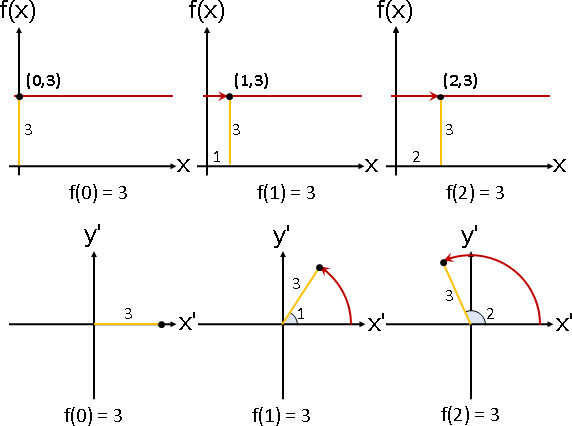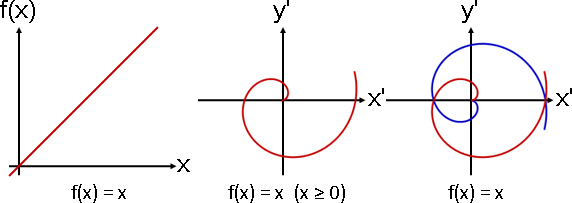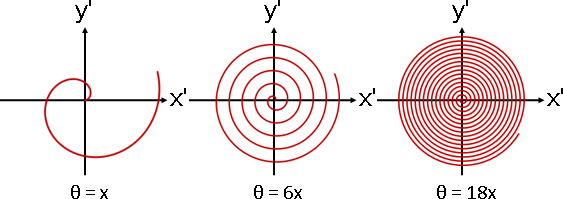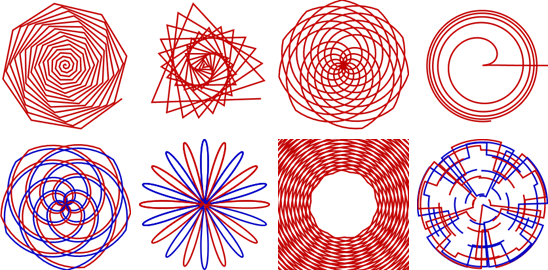Periodicity ⇨ ClosedAngle and Radius ⇨ X and F(X)【查無專有名詞】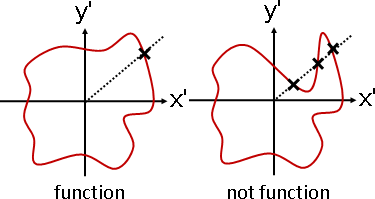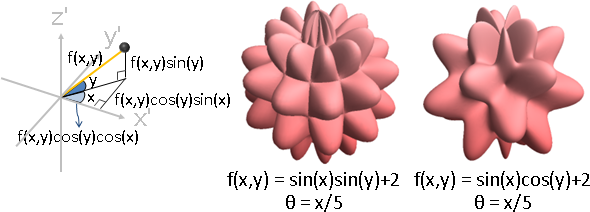f[u_,v_] := Sin[u]Sin[v]+2; ParametricPlot3D[{f[u,v]Cos[v/5]Cos[u/5], f[u,v]Cos[v/5]Sin[u/5], f[u,v]Sin[v/5]}, {u, 0, 40}, {v, 0, 40}, Boxed -> False, Axes -> False, Mesh -> None, PlotPoints -> 70, ColorFunction -> (ColorData["CherryTones"][Rescale[#3, {-2, 2}]] &) ]ParametricPlot3D[{Sin[x] Cos[x*10], Sin[x] Sin[x*10], x}, {x, 0, 9}, Boxed -> False, Axes -> False, PlotStyle -> {RGBColor[192,0,0], Thick}]

Spiral（Under Construction!）

http://www.mathematische-basteleien.de/spiral.htm

Basis Polynomial（Under Construction!）

Bernstein polynomial

```http://www.cs.jhu.edu/~misha/Spring19/
```
```https://en.wikipedia.org/wiki/Hermite_polynomials
```
```Legendre polynomial:  laplace solution of sphere coordinate
http://mathworld.wolfram.com/LaplacesEquationSphericalCoordinates.html

Hankel transform of Zernike polynomials are essentially Bessel Functions

Chebyshev polynomial: sine wave wrapped around a cylinder

[surface]

https://plot.ly/javascript/3d-surface-plots/
http://bl.ocks.org/supereggbert/aff58196188816576af0

[green function]

https://www.wolframalpha.com/input/?i=e%5E(i%7Cx-y%7C)+%2F+%7Cx-y%7C

e^(iκ|x-y|) / |x-y|

ΔG(x, y) + κ^2 G(x, y) = 0
```